Tangents and Normals (using Differentiation)

Chapter 6 Class 12 Application of Derivatives
Serial order wise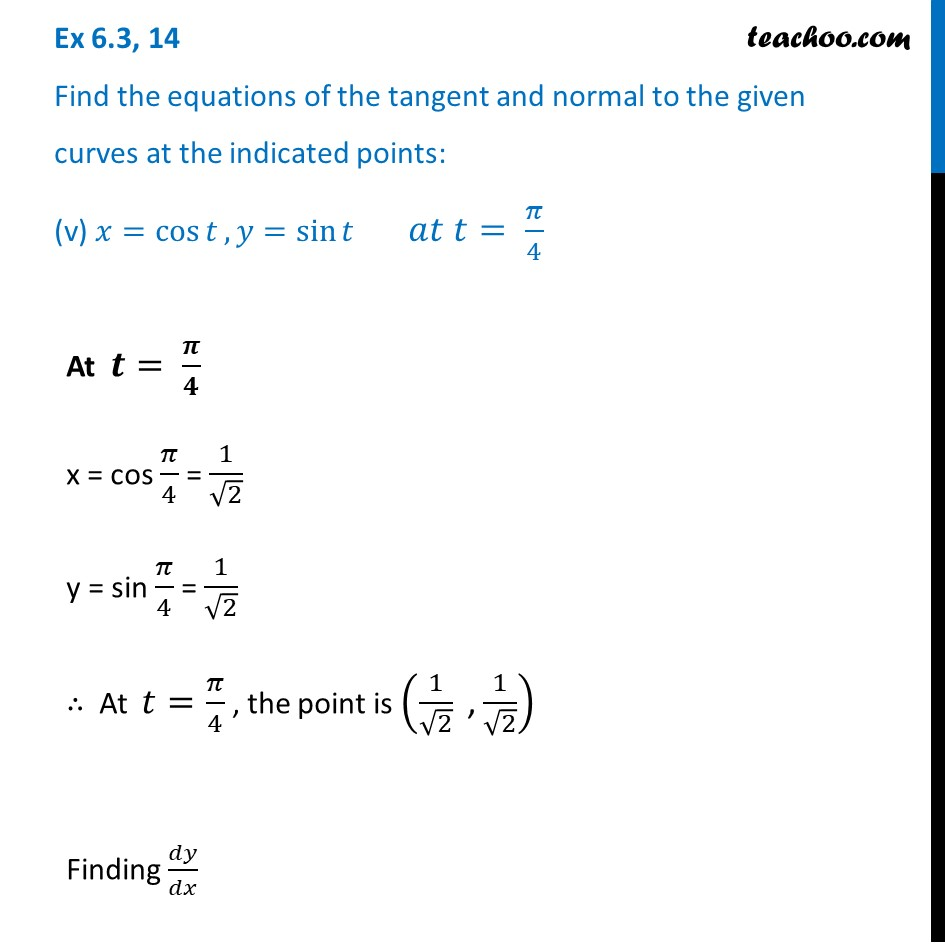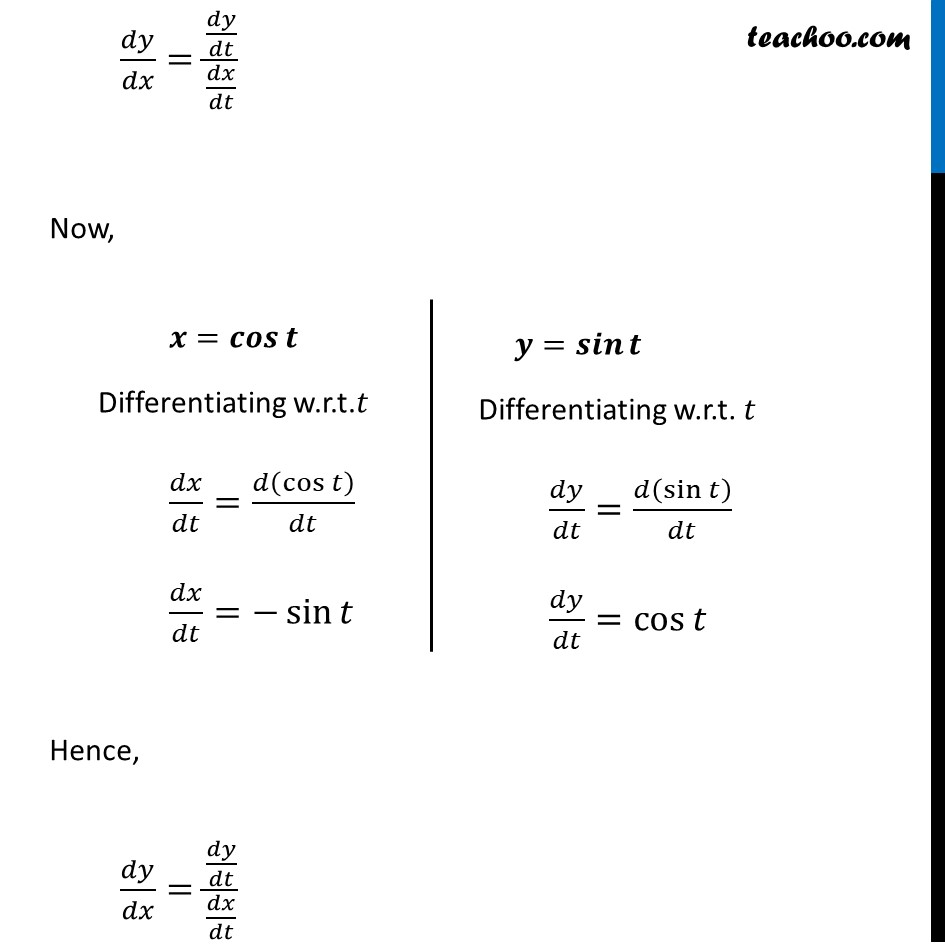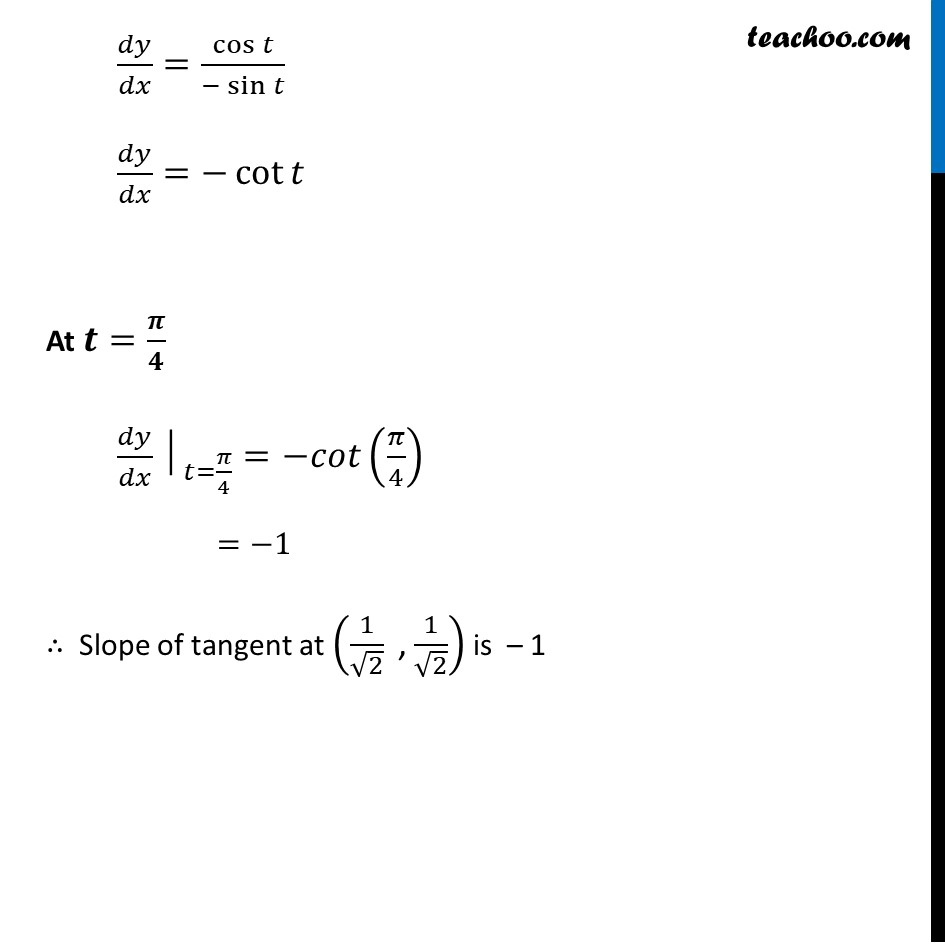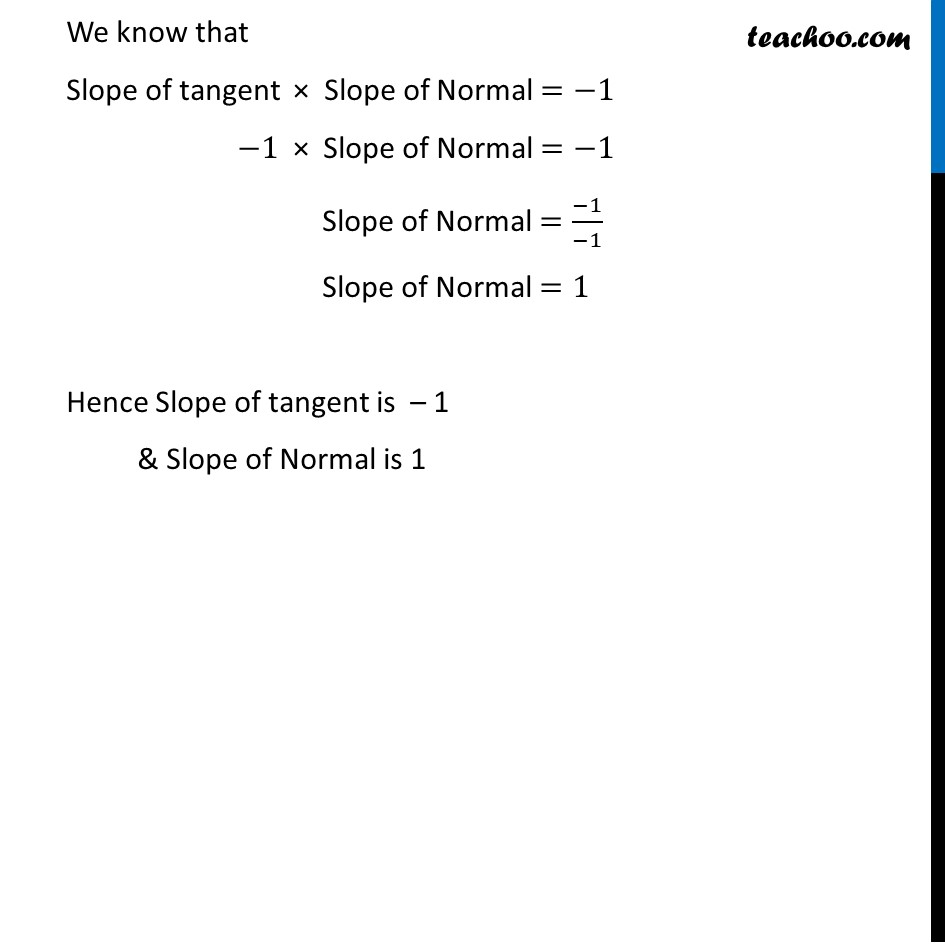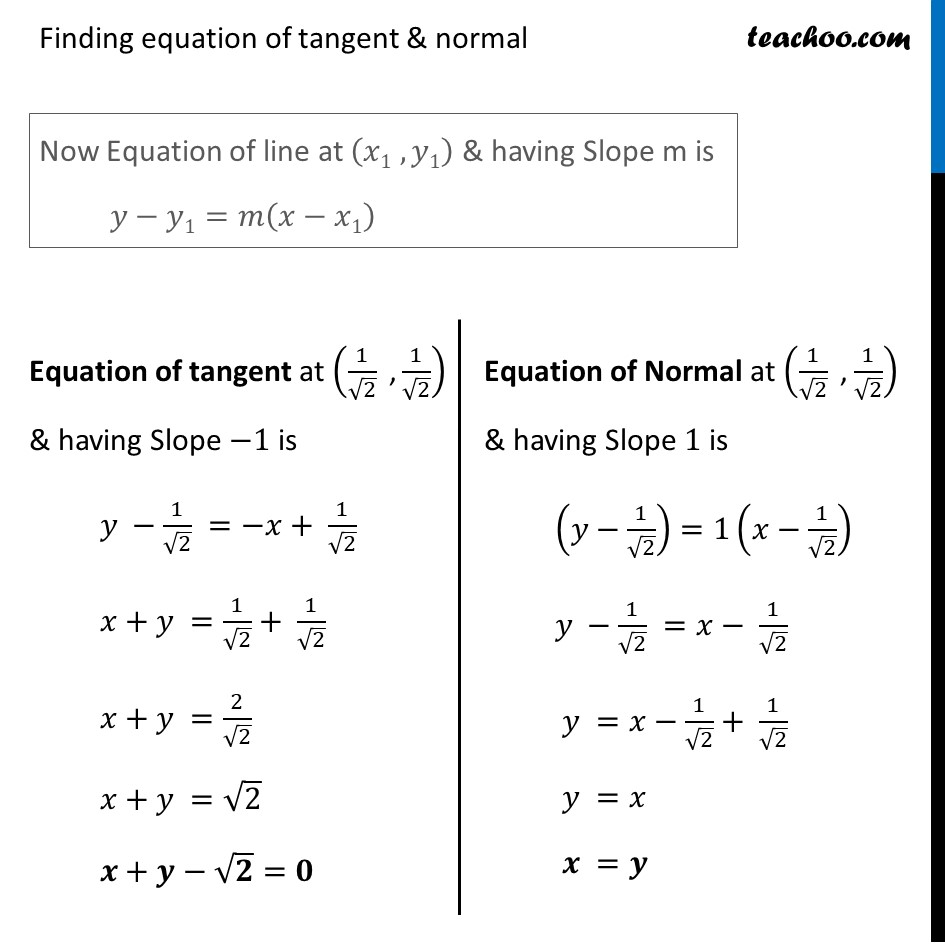Learn in your speed, with individual attention - Teachoo Maths 1-on-1 Class

### Transcript

Question 14 Find the equations of the tangent and normal to the given curves at the indicated points: (v) 𝑥=cos⁡𝑡, 𝑦=sin⁡𝑡 𝑎𝑡 𝑡= 𝜋/4At 𝒕= 𝝅/𝟒 x = cos 𝜋/4 = 1/√2 y = sin 𝜋/4 = 1/√2 ∴ At 𝑡=𝜋/4 , the point is (1/√2 " ," 1/√2) Finding 𝑑𝑦/𝑑𝑥 𝑑𝑦/𝑑𝑥=(𝑑𝑦/𝑑𝑡)/(𝑑𝑥/𝑑𝑡) Now, Hence, 𝑑𝑦/𝑑𝑥=(𝑑𝑦/𝑑𝑡)/(𝑑𝑥/𝑑𝑡) 𝒙=𝒄𝒐𝒔⁡𝒕 Differentiating w.r.t.𝑡 𝑑𝑥/𝑑𝑡=𝑑(cos⁡𝑡 )/𝑑𝑡 𝑑𝑥/𝑑𝑡=−sin⁡𝑡 𝒚=𝒔𝒊𝒏⁡𝒕 Differentiating w.r.t. 𝑡 𝑑𝑦/𝑑𝑡=𝑑(sin⁡𝑡 )/𝑑𝑡 𝑑𝑦/𝑑𝑡=cos⁡𝑡 𝑑𝑦/𝑑𝑥=cos⁡𝑡/(−sin⁡𝑡 ) 𝑑𝑦/𝑑𝑥=−cot⁡𝑡 At 𝒕=𝝅/𝟒 〖𝑑𝑦/𝑑𝑥│〗_(𝑡=𝜋/4)=−𝑐𝑜𝑡(𝜋/4) =−1 ∴ Slope of tangent at (1/√2 " ," 1/√2) is – 1 We know that Slope of tangent × Slope of Normal =−1 −1 × Slope of Normal =−1 Slope of Normal =(−1)/(−1) Slope of Normal =1 Hence Slope of tangent is – 1 & Slope of Normal is 1 Finding equation of tangent & normal Now Equation of line at (𝑥1 , 𝑦1) & having Slope m is 𝑦−𝑦1=𝑚(𝑥−𝑥1) Equation of tangent at (1/√2 " ," 1/√2) & having Slope −1 is 𝑦 −1/√2 =−𝑥+ 1/√2 𝑥+𝑦 =1/√2+ 1/√2 𝑥+𝑦 =2/√2 𝑥+𝑦 =√2 𝒙+𝒚−√𝟐=𝟎 Equation of Normal at (1/√2 " ," 1/√2) & having Slope 1 is (𝑦−1/√2)=1(𝑥−1/√2) 𝑦 −1/√2 =𝑥− 1/√2 𝑦 =𝑥−1/√2+ 1/√2 𝑦 =𝑥 𝒙 =𝒚